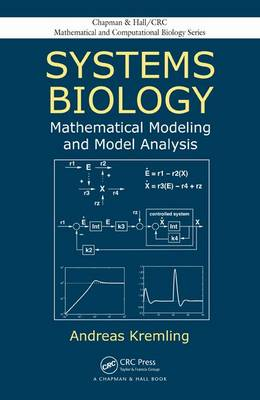•Systems Biology: Mathematical Modeling and Model Analysis - Chapman & Hall/CRC Mathematical and Computational Biology (Hardback)

(author)
£69.99
Hardback 379 Pages / Published: 12/11/2013
• We can order this

Usually dispatched within 1 week

Drawing on the latest research in the field, Systems Biology: Mathematical Modeling and Model Analysis presents many methods for modeling and analyzing biological systems, in particular cellular systems. It shows how to use predictive mathematical models to acquire and analyze knowledge about cellular systems. It also explores how the models are systematically applied in biotechnology.

The first part of the book introduces biological basics, such as metabolism, signaling, gene expression, and control as well as mathematical modeling fundamentals, including deterministic models and thermodynamics. The text also discusses linear regression methods, explains the differences between linear and nonlinear regression, and illustrates how to determine input variables to improve estimation accuracy during experimental design.

The second part covers intracellular processes, including enzymatic reactions, polymerization processes, and signal transduction. The author highlights the process-function-behavior sequence in cells and shows how modeling and analysis of signal transduction units play a mediating role between process and function.

The third part presents theoretical methods that address the dynamics of subsystems and the behavior near a steady state. It covers techniques for determining different time scales, sensitivity analysis, structural kinetic modeling, and theoretical control engineering aspects, including a method for robust control. It also explores frequent patterns (motifs) in biochemical networks, such as the feed-forward loop in the transcriptional network of E. coli.

Moving on to models that describe a large number of individual reactions, the last part looks at how these cellular models are used in biotechnology. The book also explains how graphs can illustrate the link between two components in large networks with several interactions.

Publisher: Taylor & Francis Inc
ISBN: 9781466567894
Number of pages: 379
Weight: 658 g
Dimensions: 235 x 156 x 23 mm

MEDIA REVIEWS

"Systems Biology: Mathematical Modeling and Model Analysis is a rich resource of mathematical methods and approaches that can be utilized to analyze and understand biological systems. It will be particularly attractive to engineers and mathematicians, who want to learn the basics of modern biology in a condensed fashion and then apply the tools of their trades to relevant biological questions.
Systems Biology presents key phenomena of molecular biology in a succinct, introductory manner while expecting the reader to have some prior knowledge of basic math, including probabilities, integrals, matrices, differential equations, and Laplace transforms. Building upon this knowledge, Systems Biology devotes a good portion of the material to state-of-the-art model diagnostics and engineering techniques, such as linear systems analysis and control theory, which so far are rarely found in systems biology texts and are therefore a welcome addition to the repertoire of textbook literature. For instance, important topics like identifiability, observability, and robust control are seldom encountered in introductory texts on systems biology but discussed here in some detail. Laudably, all topics are illustrated with step-by-step examples, and many of them are reinforced with exercises."

-Eberhard Voit, Georgia Institute of Technology

"Computational modeling of biological circuits, networks and pathways is one of the most exciting areas in biology today, and Andreas Kremling's Systems Biology: Mathematical Modeling and Model Analysis is a meticulous and far-reaching treatment of this critically important subject. This book clearly aims to be comprehensive without sacrificing depth, and the result is an exhaustive survey of modeling approaches. Each chapter is thoughtfully crafted to draw in novices to the field while still engaging to experts. Additionally, a number of well-designed exercises complement each chapter. I found the sections on model analysis and control theory particularly useful and relevant."

-Markus Covert, Stanford University

"[Systems Biology: Mathematical Modeling & Model Analysis] is a well-structured (the collection and order of chapters is excellent), provides comprehensive material of fundamentals, theory, and applications of methods used in systems biology. It is a user-friendly guide that I believe can serve as a tutorial for students specializing in systems biology as well as a reference work for established researchers in the field. I highly recommend this book to the reader."

-Christian T. K.-H. Stadtlander, Journal of Biological Dynamics, November 2017